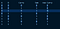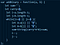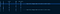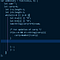# Adding Two Binary Numbers

I have found a very interesting way to add binary numbers. If we want to add binary numbers we will be needing three variables namely

1. Digit of first binary number
2. Digit of second binary number
3. Carry which we will get by adding the variables from 1 and 2.

# WHAT WE WILL DO:

1. Loop through the binary number strings and add the digits at a specific index.

2. Changing the carry whenever required

3. Return the binary after looping through all the digits of the binary number

# Explanation for STEP 1:

We take an index for both strings and start at the right most index.
Suppose we have a string a=”10001", we take an index i =a.length-1 which is equal to 4.
Similarly, if we have a string b=”001", we take an index j=b.length-1 which is equal to 2.

We take another variable as sum which stores the result of sum of the binary digits.

We take another variable carry which saves the carry after adding the digits of the binary number.

We save a[i] and b[j] in variables A and B respectively. From here on we will use A and B variables for future digits of string a and b respectively.

We start with a simple truth table for more explanation:1.0 Truth Table

here we can see the sum is the XOR of A and B and Carry

so, Sum will be equal to String(A^B^Carry) + previous sum.

I would also like to point that if A or B does not exist we change it to “0” because while looping one string may have more characters than the other string. So if A or B does not exist we change it to 0.

The code will look something like this.1.1 Looping through the digits of binary numbers

# Explanation for STEP 2:

Now we need to change the carry with respect to the sum. Lets try to use another truth table for explanation.1.2 truth table to explain when the carry will change

With the help of truth table we can check that whenever A is equal to B and carry is not equal to A or B. The new carry will change. So we now implement the same logic in our code and update carry accordingly. We add this line inside the loop.

if(A===B && A!==String(carry)){
carry=Number(!carry)
}

The code will look something like this.1.3 Updating the carry

Since, A and B are equal for this case we have compared carry in the if clause with carry. You can compare with B also. This won’t cause any issue.

# Explanation for STEP 3:

Now we have our sum updated. Only thing that remains is to return the sum.
If we have a carry with value equal to 1. we will return carry as a string plus sum otherwise we will return sum.

return carry?String(carry)+sum:sum;

So the final code will look something like this:

So here is how you add two binary numbers.

Let me know about your thoughts. Thank you

I am a Front-End Developer who loves to travel and solving algorithms

## More from Kshitiz

I am a Front-End Developer who loves to travel and solving algorithms